# CBSE Class 3 Maths Sample Paper Set K

Read and download PDF of CBSE Class 3 Maths Sample Paper Set K designed as per the latest curriculum and examination pattern for Class 3 issued by CBSE, NCERT and KVS. The latest Class 3 Mathematics Sample Papers have been provided with solutions so that the students can solve these practice papers and then compare their answers. This will help them to identify mistakes and improvement areas in Mathematics Standard 3 which they need to study more to get better marks in Grade 3 exams. After solving these guess papers also refer to solved Class 3 Mathematics Question Papers available on our website to build strong understanding of the subject

## Mathematics Sample Paper Class 3

Students can refer to the below Class 3 Mathematics Sample Paper designed to help students understand the pattern of questions that will be asked in Grade 3 exams. Please download CBSE Class 3 Maths Sample Paper Set K

### Mathematics Class 3 Sample Paper

CBSE Class 3 Maths Sample Paper Set K .Sample Papers are the very important for every student. The sample papers should be practiced to gain extra marks in examinations. The sample papers have been prepared based on summative assessment1 and summative assessment 2 pattern. The sample papers have been prepared based on pattern of last year examinations and as per latest changes in the syllabus. Students, teachers and parents can download all CBSE educational material and very well prepared worksheets from this website.  All CBSE educational material is developed by our panel of teachers, have also been submitted by CBSE teachers and students.

I. Choose the correct answer and fill in the blanks. (½ x 10 = 5)

a) 4 times 9 is written as ______________ (4 + 9 , 4 x 9 , 4 ÷ 9)

b) It takes _____________ to set curds. (days hours minutes )

c) 30 + 30 + 30 = _____ x 30 = 90 (30 3 90)

d) 16 ÷ 2 = _________ (8 14 4)

e) Of the following things _______________ can hold a 1000 litres of water.(a bucket, a water tank, an eye dropper)

f)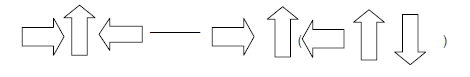g) The short hand in a clock is called the ___________ hand.(hour minute second )

h) h) 8000 millilitre = _______ litres. (80 800 8)

i) The shortest month of a year is ________________.(March January February)

j) The time shown by the hands on the clock is ___________.(two fifteen, three thirty , three ten)\

II A. Fill in the blanks: (½ × 5 = 2 ½)

a) ½ litre = _____________ millilitre

b) 1 day = ____________ hours

c) 3 x _____ = 18

d) 3 , 8 , 13 , ______B. Match the following. (½ × 5 = 2½)

a) 6 + 6 + 6 + 6 Re 1

b) 18 ÷ 9 multiples of 2

c) 4 and 6 6 x 4

d) 7 x 8 2

e) Two 50 paise makes 56

III. Do as directed. (1 × 10 = 10 )

a) Draw the hands for the given time.b) Find the secret message.

1 2 I 2 3       3 4 A 4 5 M 5 6         6 7 F 7 8 I 8 9 N 9 1 E 1 2

______________________________________________________

c) Write all the even numbers between 502 and 507.

_______________________________________________________

d) How much money does it make?

Two fifty rupee notes = Rs ________________

e) How many corners would four triangles have?

_______________________________________________________

f) Complete the following.

30, 60, 90, ______________ , _____________

g) Find out the rule and continue the patterns.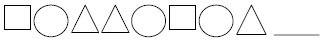h) Write the following in alphabetical order.

Arya Chitra Akash Himanshu

_______________________________________________________

i) A frog jumps 4 steps at a time.It reaches 20 in ______________ jumps.

j) Four times 5 is written as ________ = ________

IV. Do as directed. (1 × 10 = 10)

a) Two and half dozen bananas are equal to how many bananas?

b) Write in figures.

Six rupees and fifty paise - _____________

c) Write the missing multiples.d) If we add 1 to any odd number then we get an ______________ number.

e) There are 28 buttons in 7 shirts.

There are ______________ buttons in one shirt.

f) Complete the pattern.

1A, 2B, 3C, _______, 5E

g) Subtract

9    l    300 ml

3    l   200 ml

________________

________________

i) The odd number between 16 and 18 is ___________.

Rs 2 5 . 5 0

+ Rs 6 1 . 0 0

___________

___________

V. SOLVE (2 × 10 = 20)

a) Look at the calendar and answer the following questions.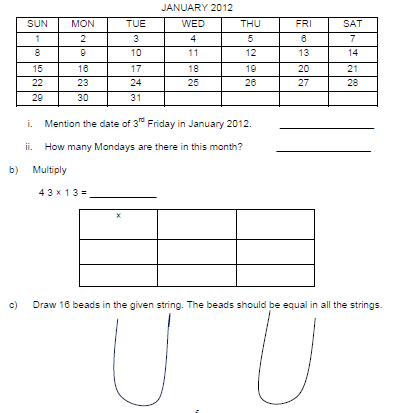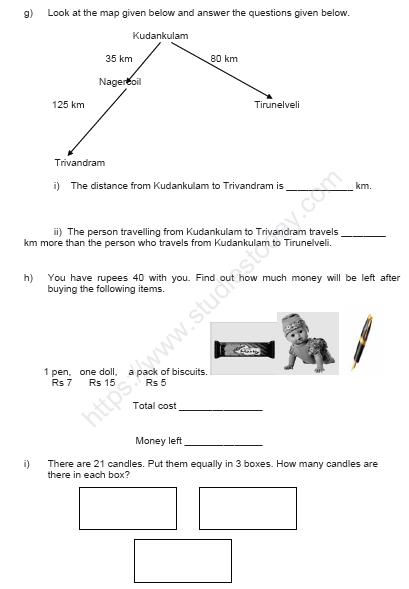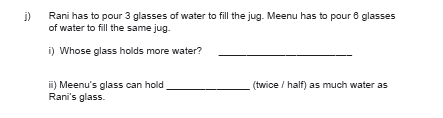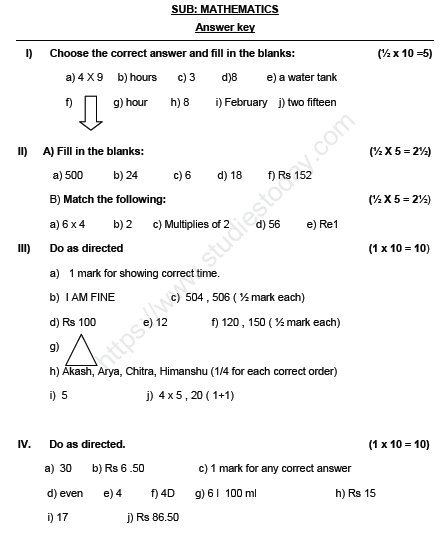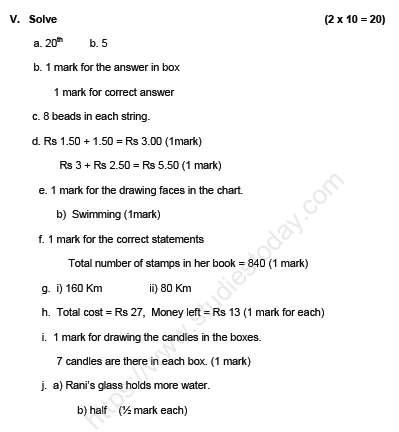Please click the below link to access CBSE Class 3 Maths Sample Paper Set K

## Tags:

Click for more Mathematics Study Material
 CBSE Class 3 Maths Sample Paper Set L CBSE Class 3 Maths Sample Paper Set D CBSE Class 3 Maths Sample Paper Set N CBSE Class 3 Maths Sample Paper Set C CBSE Class 3 Maths Sample Paper Set K CBSE Class 3 Maths Sample Paper Set B CBSE Class 3 Maths Sample Paper Set A CBSE Class 3 Maths Sample Paper Set I CBSE Class 3 Maths Sample Paper Set E CBSE Class 3 Maths Sample Paper Set G CBSE Class 3 Maths Sample Paper Set M CBSE Class 3 Maths Sample Paper Set H CBSE Class 3 Maths Sample Paper Set F CBSE Class 3 Maths Sample Paper Set J

## Latest NCERT & CBSE News

Read the latest news and announcements from NCERT and CBSE below. Important updates relating to your studies which will help you to keep yourself updated with latest happenings in school level education. Keep yourself updated with all latest news and also read articles from teachers which will help you to improve your studies, increase motivation level and promote faster learning

### How To Solve Unseen Passages In English

Unseen passages may contain one or many paragraphs. This is one of the important yet easy parts for a student to get marks. Students should thoroughly study and understand the passage to answer the related questions. The unseen passages are there just to test the...

### CBSE Class 10 Revised Syllabus

Last year CBSE had to reduce the syllabus because of the pandemic situation but it was not very effective because there were no examinations. This year to avoid any confusion and conflict, CBSE has decided to reduce the syllabus into term 1 and term 2. 50 percent of...

### MCQ Question based CBSE examination

For 2021-22 CBSE has launched MCQ question-based examination for Term 1 & Term 2 board examinations. The entire syllabus has been divided into two parts each including 50% of the entire syllabus. To score well, students must practice as per the new CBSE term-wise...

### Score well in Class 12 English Boards Exam

12th Board exams are an important part of students' lives. The marks obtained in the board exam decide the college in which one can study. In class 12 the syllabus of each and every subject increases vastly and it is difficult to cover up every point. In English also...

×